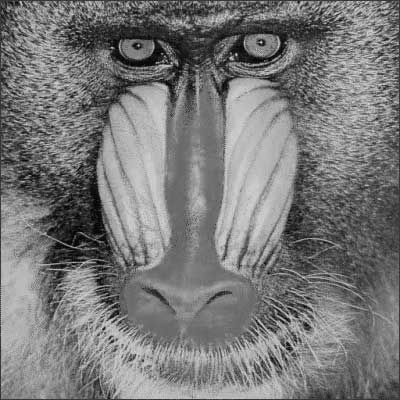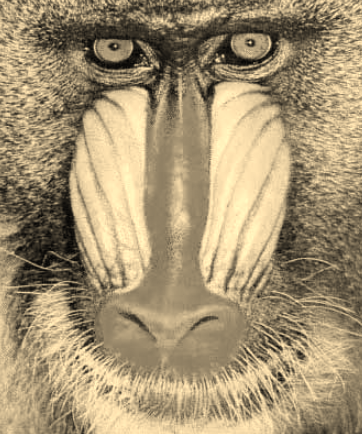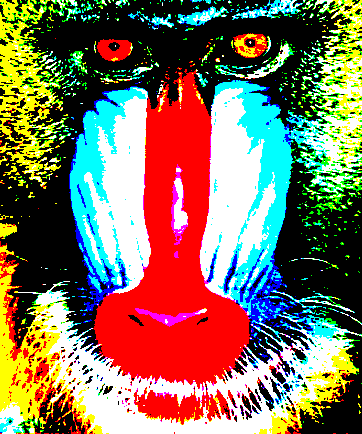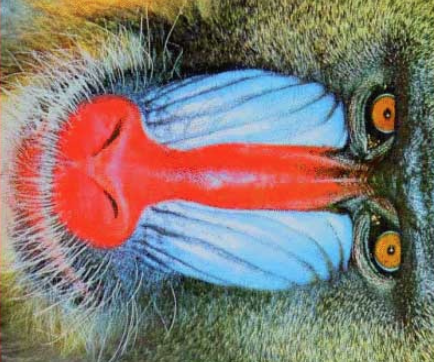## Homework 25 — image manipulation

Due Wednesday November 28

### Modules color.py and locate.py

• In classes 34 and 35 we investigated the power of patterns and made use of them to simplify image manipulation. In these classes we considered and developed modules
• Provides a pattern for photo manipulation and a collection of convenience fuctions to support blueing, color inversion, flipping, mirroring, mono coloring, greyscaling, sepia toning, and palette reduction.
• Provides functions to return the dimensions of two types of image manipulation — same size and clockwise rotation
• Provides functions for determining where is the source pixel for a pixel manipulation supported by `manip.py`
• Provides functions for the pixel manipulations supported by `manip.py`
• The task at hand is to implement module `color.py` functions: `greyscale()`, `sepia_tone()` and `reduce_palette()` and module `locate.py` function `ccw()`.

### Grayscaling — `color.py` function `grey( p )`

• In converting an image to its grayscale equivalent the translation sacrifices pixel coloring for intensity. In the conversion, a pixel `p` equal to `(r,g,b)` corresponds to a pixel `(m,m,m)` where `m` is the integer cast of

.299r + .587g + .114b

The formula is known as the NTSC formula.### Sepia — `color.py` function `sepia( p )`

• There is no one formula for defining a Sepia pixel conversation. The one used here comes from Microsoft.
• For pixel `(r,g,b)`, the Sepia conversion is `(sr,sg,sb)` where

sr, sg, sb = int( min( i, 255 )), int( min( j, 255 )), int( min( k, 255 )),

where `i, j, k` are

i = .393r + .769g + .189b

j = .349r + .686g + .168b

k = .272r + .534g + .131b### Palette reduction — `color.py` functions `distance( p )` and `simplify( p )`

• Sometimes to save computer memory, software developers perform a palette reduction of colors used in image representation.
• Our reduction scheme will use an eight-color palette
• Black, White, Red, Green, Blue, Yellow, Magenta, and Cyan; that is, `(0,0,0), (255,255,255), (255,0,0), (0,255,0), (0,0,255), (255,255,0), (255,0,255),` and `(0,255,255)`
• In a palette reduction, a pixel `(r,g,b)` is replaced by the pixel in in the palette it is closest to. The distance between pixels `(r1,g1,b1)` and `(r2,g2,b2)` equals

abs(r1 - r2) + abs(g1 - g2) + abs(b1 - b2)### Clockwise rotation `locate.py` function `ccw( spot, w, h )`

• In a clockwise rotation an image is rotated by 90 degrees.